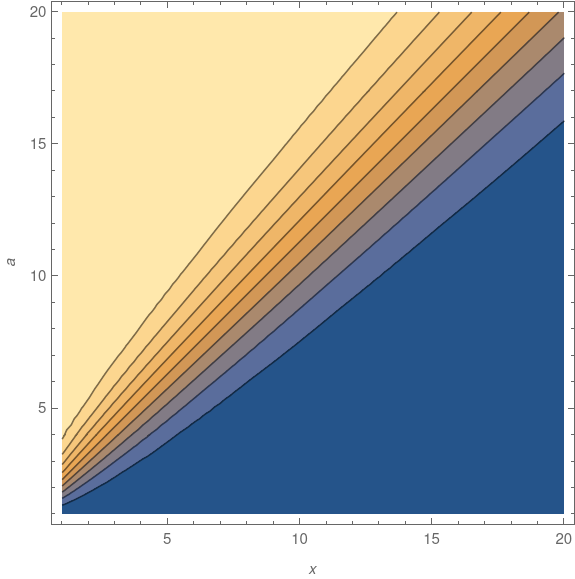# Gamma distribution tail probability approximation

This post will approximate of the tail probability of a gamma random variable using the heuristic given in the previous post.

## The gamma distribution

Start with the integral defining Γ(a). Divide the integrand by Γ(a) so that it integrates to 1. This makes the integrand into a probability density, and the resulting probability distribution is called the gamma distribution with shape a. To evaluate the probability of this distribution’s tail, we need to integrateThis distribution has mean and variance a, and mode a-1.

We’re interested in approximating the integral above for moderately large x relative to a.

## Approximation

To apply the 1/e heuristic we need to find a value of t such that

ta-1 exp(-t) = xa-1 exp(-x)/e = xa-1 exp(-x – 1).

This equation would have to be solved numerically, but lets try something very simple first and see how it works. Let’s assume the ta-1 term doesn’t change as much as the the exp(-t) term between x and x + 1. This says an approximate solution to our equation for t is simply

t = x + 1

and so the base of our rectangle runs from x to x + 1, i.e. has width 1. So the area of our rectangle is simply its height. This gives usIn other words, the CDF is approximately the PDF. That’s all nice and simple, but how good is it? Here’s a plot for a = 5.So is this good or not? It’s not a question of whether but of which. It’s a poor approximation for some parameters but a very good approximation for others. The approximation improves when a is closer to 1 and when x is larger.

We were kinda sloppy in solving for t above. Would it help if we had a more accurate value of t? No, that doesn’t make much difference, and it actually makes things a little worse. The important issue is to identify over what range of a and x the approximation works well.

## Asymptotic series

It turns out that our crude integration estimate happens to correspond to the first term in an asymptotic series for our integral. If X is a gamma random variable with shape a then we have the following asymptotic series for the tail probability.So if we truncate the portion inside the parentheses to just 1, we have the approximation above. The approximation error from truncating an asymptotic series is approximately the first term that was left out.

So if x is much larger than a, the approximation error is small. Since the expected value of X is a, the tail probabilities correspond to values of x larger than a anyway. But if x is only a little larger than a, the approximation above won’t be very good, and adding more terms in the series won’t help either.

Here’s a contour plot of the absolute error:The deep blue area below the diagonal of the graph shows the error is smallest when x > a. In 3D, the plot has a high plateau in the top left and a valley in the lower right, with a rapid transition between the two, suggested by the density of contour lines near the diagonal.

## Epilogue

In the previous post I said that the next post would give an example where the 1/e heuristic doesn’t work well. This post has done that. It’s also given an example when the heuristic works very well. Both are the same example but with different parameters. When x >> a the method works well, and the bigger x is relative to a, the better it works.